# Geometría proyectiva: Projective center of two projective bundles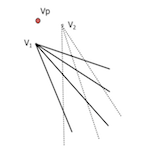Using the laws of duality in projective models can get a set of properties and dual theorems from other previously deducted. Obtaining homologous elements in the projective case series was performed by obtaining intermediate pespectividades allowed perspectival do we get what we have called “projective axis”. We will see that in the case of projective bundles, Dual reasoning leads us to determine projective centers.

The “world” points is more affordable than didactically straight dual, so we have started the analysis with the concepts associated with rectilinear series for, now, carry out development in dual forms, straight beams.

We can consider in this study a series of basic questions that will help guide the development:

• How do we define two projective?
• How many homologous elements are necessary to determine a projectivity
• How can we obtain homologous elements from given?

Two projective bundles are determined to define three pairs of homologous straight (a-a’, b-b’, c-c’), located on their respective bases (originating in their respective vertices).

A fourth element beam vertex x “V1” shall have one new ray x’ homologue beam (projective) base (vertex) “V1”” so that the cross ratio of quaternions is retained determining:

(abcx) = (a’b’c’x’)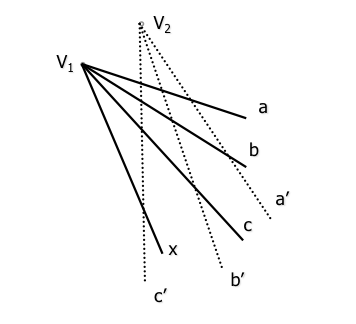To determine the homologue of x will operate using intermediate between sets that relate perspectividades (sectioned) elements of both beams.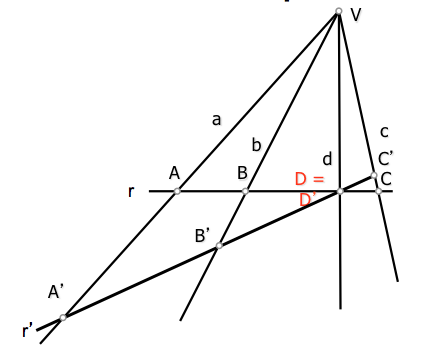By studying the perspectivity we saw that series of perspectives (perspective center with a common vertex hazproyección), have a double point that is contained in the base (intersection of the straight brackets) series.

In the figure the double point is D = D’ containing a straight r and r’ series of perspectival perspectival center point with V.

This property is essential for finding prospects series linking the two projective bundles which means simplified treatment, as discussed below.

Given two projective bundles freely V1 and V2, proceed to cut them by two lines r and r’ determining series with those prospects that are doing. Among the countless pairs of lines that we can use to sever these beams, choose two passing through any point of intersection of two homologous elements of the beams. The point D = D’ contains the pair d-d’ of these beams.

These series of points of bases r and r 'are perspectival each being double point D = D '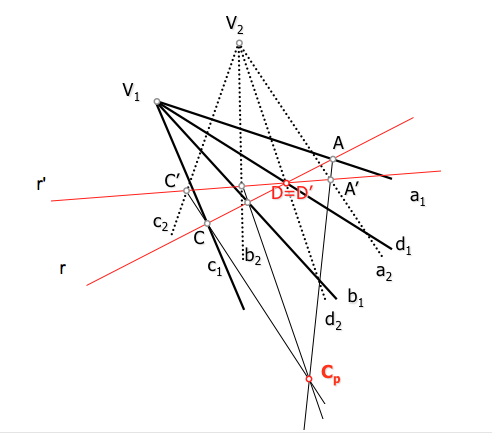Point Cp is the perspective center of the series de bases r and r’ that section of the beam rays. Varying any of the bases of the series (r o r’) on section D-D ', this series will continue to be prospects (having a double point) but the perspective changes from center position. Although the center change, construction for determination of homologous elements remain equally valid.

### Projective Center

By using two beams as bases homologous series of r and r ', these perspectives to have a dual element. We are in the previous case because the bases are passing by a point (D-D’) containing two homologous elements, but in this case the perspective center of the series is unique and depends on the selected pair of rays to generate perspectives series. If so we cut from a-a’ o b-b’ … the perspective center is the same and call it “projective center beams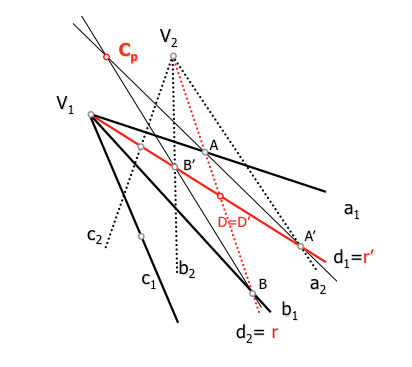The point is Cp center prospective series de bases r y r’, being in turn the projective center beams de bases V1 y V2

Rays m = n’ projection of the two bases (rectas V1-V2) have peer-projection from the center of the corresponding bases.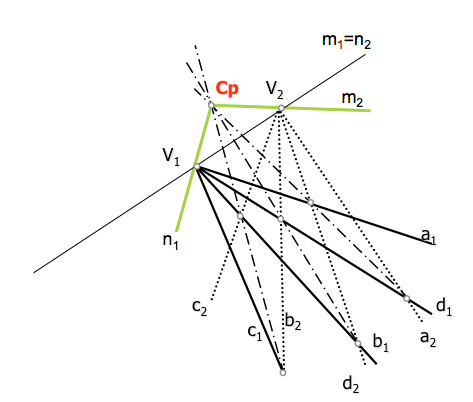We will see later how to use the projective center to determine pairs of homologous elements of the beams.